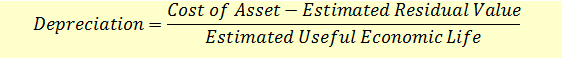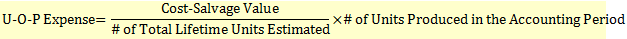# Depreciation Methods for Property, Plant, and Equipment (PPE)

Depreciation is defined as the ‘allocation of the depreciable amount of an asset over its estimated life’. According to the matching concept, revenues should be matched with expenses in order to determine the accounting profit. The cost of the asset purchased should be spread over the periods in which the asset will benefit a company.

We will study the following methods of depreciation:

• Straight-line Method
• Accelerated Depreciation Methods
• Sum-of-the-Years Digits (SYD)
• Double-declining Balance (DDB)
• Units-of-production Method

### Straight-line Method

The straight-line method associates the long-lived asset’s usefulness with its age. Under this method, depreciation is computed by dividing the depreciable amount of the asset by the expected number of accounting periods of its useful life.Note that useful economic life is not equal to physical life. It is the period over which the firm intends to use the asset.

Residual amount is the amount received after disposal of the asset.

Example

Cost of Asset = $1,000 Residual/Salvage value =$200

Estimated Useful life = 4 years

Annual charge for depreciation = (1,000 – 200)/4 = $200 ### Accelerated Depreciation Methods Accelerated depreciation methods allow higher depreciation to be charged in the early years, and lower depreciation in the later periods. The two common accelerated depreciation methods are: Sum-of-the-Years Digits (SYD) expensing and Double Declining Balance (DDB) expensing. ### Sum-of-the-Years Digits (SYD) SYD method treats an asset as more useful in its early life by raising the depreciation expense for the early years. SYD Example: If a company’s factory has a new conveyor belt with a useful life of 5 years, then SYD = 1+2+3+4+5 = 15. This conveyor belt cost$100,000 and has a salvage value = $0. The year two depreciation expense under the SYD method for the company will be calculated as follows: ($100,000 – $0) * (5 – 2 +1)/15 =$100,000*(4/15) = $26,667 SYD Depreciation Expense for Year “i” = (Cost – Salvage Value) * ((n – “# of the ith year” +1))/SYD ### DDB Methods DDB method accelerates the depreciation rate of the straight line method. DDB Expense = (Cost – Accumulated Depreciation) * (2/n) Example Cost of Asset =$1,000

Estimated Useful life = 4 years

DDB depreciation in year 1 = 1,000*2/4 = 500

DDB depreciation in year 2 = (1,000 – 500)*2/4 = $250 And so on… Note that the DDB depreciation calculation does not include salvage/residual value. When the book value of the asset reaches its salvage value, no more depreciation expense will be recognized. ##### Units-of-production Method Unlike the time based methods of straight line and accelerated depreciation, the Units-of-Production (U-O-P) depreciation method is activity based. A year’s depreciation expense on an annual income statement will include that year’s production as a fraction of total estimated lifetime production from the asset.Example A company bought a machine at$10,000 and expects that the machine would run for 2,000 hours during its life.  It is expected to have no scrap value. The machine was run 800 hours in year 1, 600 hours in year 2, 350 hours in year 3, and 250 hours in year 4. Assuming no salvage value, the depreciation charge will be calculated as follows:

Year 1: $10,000 * 800/2,000 =$4,000

Year 2: $10,000 * 600/2,000 =$3,000

Year 3: $10,000 * 350/2,000 =$1,750

Year 4: $10,000 * 250/2,000 =$1,250

Once a company has invested in a long-lived asset, it must:

• Choose a depreciation method;
• Estimate the useful life of the asset over which the depreciation will take place; and
• Determine if the asset will have a salvage value at the end of its depreciable life.